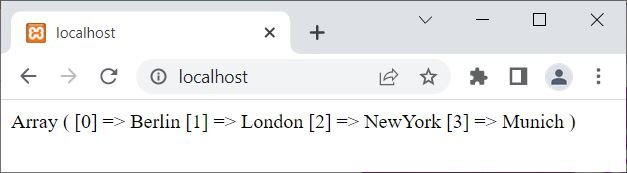# PHP print_r() function

The PHP "print_r()" function is used to print information of a specified variable and looks more readable. Or we can say that the print_r() function is used to print an array, object, or variable's contents in a human-readable format. Debugging and comprehending the composition and contents of complex data types are facilitated by it. For example:

```<?php
\$x = array("Berlin", "London", "NewYork", "Munich");
print_r(\$x);
?>```

The output of the above PHP example on the print_r() function is:See the output of \$x, an array variable. We can clearly see that, at index 0, the Berlin is stored. Similarly, at index 1, London is stored, and so on. That is, if you use the following PHP statement:

`echo \$x;`

Then you will get Berlin as an output.

## PHP print_r() syntax

The syntax of the print_r() function in PHP is:

`print_r(variable, return)`

The first parameter (variable) is required, whereas the second parameter (return) is optional.

The "variable" parameter is used to specify the variable about which we want to print information.

Note: The default value of the return parameter is false. But if we specify this parameter as true, then the function print_r() returns the information without printing.

Note: If the variable parameter is an integer, float, or string type value, then the function print_r() prints the value itself. Whereas if the variable parameter is an array or an object, then it returns the information about the variable in the form of keys and elements.

### Advantages of the print_r() function in PHP

• Simple and straightforward to use: print_r() can be used to quickly print the contents of an array or object.
• Human-readable output: The output of print_r() is formatted to be simple to read and comprehend for people. This makes it an excellent tool for troubleshooting and comprehending intricate data structures.
• print_r() is capable of handling nested arrays and objects, which can be challenging to print out using other techniques.

### Disadvantages of the print_r() function in PHP

• print_r() only prints the contents of an array or object, which limits its functionality. It does not offer any extra features, like sorting or filtering.
• Not recommended for large data sets: print_r()'s output can quickly become overwhelming for very large data sets, making it difficult to read and understand.
• Security issues: If print_r() is used incorrectly, it could give hackers access to confidential data. As a result, it shouldn't be used in production code and should only be used for debugging.

PHP Online Test

« Previous Tutorial Next Tutorial »